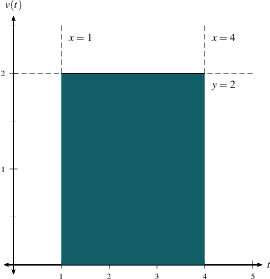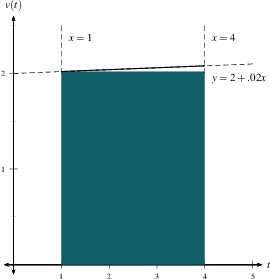Home

#### The Six Pillars of Calculus

A picture is worth 1000 words

#### Trigonometry Review

The basic trig functions
Basic trig identities
The unit circle
Addition of angles, double and half angle formulas
The law of sines and the law of cosines
Graphs of Trig Functions

#### Exponential Functions

Exponentials with positive integer exponents
Fractional and negative powers
The function $f(x)=a^x$ and its graph
Exponential growth and decay

#### Logarithms and Inverse functions

Inverse Functions
How to find a formula for an inverse function
Logarithms as Inverse Exponentials
Inverse Trig Functions

#### Intro to Limits

Close is good enough
Definition
One-sided Limits
How can a limit fail to exist?
Infinite Limits and Vertical Asymptotes
Summary

#### Limit Laws and Computations

A summary of Limit Laws
Why do these laws work?
Two limit theorems
How to algebraically manipulate a 0/0?
Limits with fractions
Limits with Absolute Values
Limits involving Rationalization
Limits of Piece-wise Functions
The Squeeze Theorem

#### Continuity and the Intermediate Value Theorem

Definition of continuity
Continuity and piece-wise functions
Continuity properties
Types of discontinuities
The Intermediate Value Theorem
Examples of continuous functions

#### Limits at Infinity

Limits at infinity and horizontal asymptotes
Limits at infinity of rational functions
Which functions grow the fastest?
Vertical asymptotes (Redux)
Toolbox of graphs

#### Rates of Change

Tracking change
Average and instantaneous velocity
Instantaneous rate of change of any function
Finding tangent line equations
Definition of derivative

#### The Derivative Function

The derivative function
Sketching the graph of $f'$
Differentiability
Notation and higher-order derivatives

#### Basic Differentiation Rules

The Power Rule and other basic rules
The derivative of $e^x$

#### Product and Quotient Rules

The Product Rule
The Quotient Rule

#### Derivatives of Trig Functions

Two important Limits
Sine and Cosine
Tangent, Cotangent, Secant, and Cosecant
Summary

#### The Chain Rule

Two forms of the chain rule
Version 1
Version 2
Why does it work?
A hybrid chain rule

#### Implicit Differentiation

Introduction and Examples
Derivatives of Inverse Trigs via Implicit Differentiation
A Summary

#### Derivatives of Logs

Formulas and Examples
Logarithmic Differentiation

In Physics
In Economics
In Biology

#### Related Rates

Overview
How to tackle the problems

#### Linear Approximation and Differentials

Overview
Examples
An example with negative $dx$

#### Differentiation Review

Basic Building Blocks
Product and Quotient Rules
The Chain Rule
Combining Rules
Implicit Differentiation
Logarithmic Differentiation
Conclusions and Tidbits

#### Absolute and Local Extrema

Definitions
The Extreme Value Theorem
Fermat's Theorem
How-to

#### The Mean Value and other Theorems

Rolle's Theorems
The Mean Value Theorem
Finding $c$

#### $f$ vs. $f'$

Increasing/Decreasing Test and Critical Numbers
How-to
The First Derivative Test
Concavity, Points of Inflection, and the Second Derivative Test

#### Indeterminate Forms and L'Hospital's Rule

What does $\frac{0}{0}$ equal?
Indeterminate Differences
Indeterminate Powers
Three Versions of L'Hospital's Rule
Proofs

Strategies
Another Example

#### Newton's Method

The Idea of Newton's Method
An Example
Solving Transcendental Equations
When NM doesn't work

#### Anti-derivatives

Anti-derivatives and Physics
Some formulas
Anti-derivatives are not Integrals

#### The Area under a curve

The Area Problem and Examples
Riemann Sums Notation
Summary

#### Definite Integrals

Definition
Properties
What is integration good for?
More Examples

#### The Fundamental Theorem of Calculus

Three Different Quantities
The Whole as Sum of Partial Changes
The Indefinite Integral as Antiderivative
The FTC and the Chain Rule

### What is integration good for?

As we have seen, definite integrals can be used to compute area, just as derivatives can be used to compute slopes of tangent lines. But there is much more to derivatives than slopes of tangent lines, and there is much more to integrals than area. Integrals can be used to compute any bulk quantity.

 To compute any bulk quantity: Break it up into small pieces (of size $\Delta x$) that are easier to understand. Estimate the contribution $f(x)\, \Delta x$ of each piece. Add up the pieces to get the Riemann Sum $\sum_{i=1}^n f(x_i^*) \,\Delta x$. Take a limit as you slice finer and finer to get the exact answer: $$\int_a^b f(x) \,dx = \lim_{N\to\infty} \,\sum_{i=1}^n f(x_i^*) \,\Delta x.$$

#### Example: Distance

Suppose that we know a particle's velocity as a function of time. If the velocity is constant, then it's easy to find the distance traveled: $$\hbox{Rate} \times \hbox{Time} = \hbox{Distance}.$$ If the velocity is almost constant, then we can get a good approximation by pretending that it is constant. If the velocity varies a lot, then we break our time interval into little sub-intervals on which the velocity doesn't change much, estimate each one, and add up the pieces. This is exactly the same strategy that we used for area under a curve.

 Example 1: Find the distance traveled by a person walking at 2 MPH from 1:00 PM to 4:00 PM.Solution: 3 hours at 2 MPH makes $3 \times 2 = 6$ miles.

 Example 2: Find the approximate distance traveled by a person walking at velocity $v(t)=2 + 0.02 t$ MPH between $t=1$ (o'clock) and $t=4$ (o'clock).Solution: The velocity doesn't change much between 1 and 4 PM, so we approximate our velocity as $v(1)=2.02$ and compute $(4-1)v(1) = 3(2.02)=6.06$ miles traveled.

The answer in Example 2 is a little bit too small, since the velocity goes up from $v=2.02$ at $t=1$ to $v=2.08$ at $t=4$. If we wanted an overestimate of the area, we would use the value of $v(t)$ at the right endpoint instead: $(4-1)v(4)=3(2.08)=6.24$. And if we wanted a more accurate guess, we might use the midpoint $f(2.5)=2.05$ and estimate the area as $(4-1)v(2.5)=3(2.05)=6.15$. But whether we use the left endpoint, the right endpoint, or the midpoint, we get roughly the same answer, since the velocity just isn't changing very much between $t=1$ and $t=4$.

By now you've probably noticed that this is exactly like finding the area under $y= 2 + 0.02x$ between $x=1$ and $x=4$. Only, in this case, the function is $v$ instead of $f$, and the variable $t$ instead of $x$, but it's the same calculation.

In general,

 To compute the distance traveled between time $t=a$ and time $t=b$ from the function $v(t)$, we Break our time interval into $n$ pieces, so that the velocity is practically constant in each piece. The distance traveled in the $i$-th time interval is approximately $v(t_i^*)\Delta t$. Add these up and take a limit to get a total distance of $$\int_a^b v(t) dt = \lim_{n\to\infty} \sum_{i=1}^n v(t_i^*) \Delta t.$$